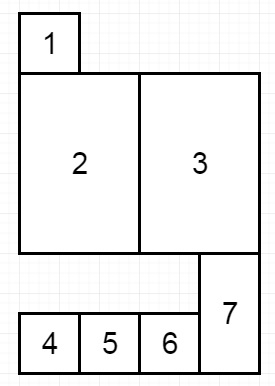# Filling Bookcase Shelves in Python

Suppose we have a sequence of books − Here the i-th book has thickness books[i] and height books[i]. If we want to place these books in order onto bookshelves that have total width shelf_width. If we choose some of the books to place on this shelf (such that the sum of their thickness is <= shelf_width), then build another level of shelf of the bookcase where the total height of the bookcase has increased by the maximum height of the books we can put down. We will repeat this process until there are no more books to place. We have to keep in mind that at each step of the above process, the order of the books we place is the same order as the given sequence of books. We have to find the minimum possible height that the total bookshelf can be after placing shelves in this manner. So if the input is like − [[1,1], [2,3], [2,3], [1,1], [1,1], [1,1], [1,2]], and the self_width = 4,then the output will be 6 as the sum of the heights of the 3 shelves are 1 + 3 + 2 = 6. Notice that book number 2 does not have to be on the first shelf.

To solve this, we will follow these steps −

• Create one array dp whose size is same as books, and fill this using infinite
• dp := books[0,1]
• for i in range 1 to length of books – 1
• curr_height := 0
• temp := self_width
• j := i
• while j >= 0 and temp – books[j, 0] >= 0, do
• curr_height := max of books[j, 1], curr_height
• dp[i] := min of dp[i], curr_height + (dp[j - 1] if j – 1 >= 0, otherwise 0)
• temp := temp – books[j, 0]
• decrease j by 1
• return last element of dp

Let us see the following implementation to get better understanding −

## Example

class Solution(object):
def minHeightShelves(self, books, shelf_width):
"""
:type books: List[List[int]]
:type shelf_width: int
:rtype: int
"""
dp = [float('inf') for i in range(len(books))]
dp = books
for i in range(1,len(books)):
current_height = 0
temp = shelf_width
j = i
while j>=0 and temp-books[j]>=0:
current_height = max(books[j],current_height)
dp[i] = min(dp[i],current_height +( dp[j-1] if j-1 >=0 else 0))
temp-=books[j]
j-=1
#print(dp)
return dp[-1]

## Input

[[1,1],[2,3],[2,3],[1,1],[1,1],[1,1],[1,2]]
4

## Output

6